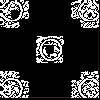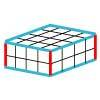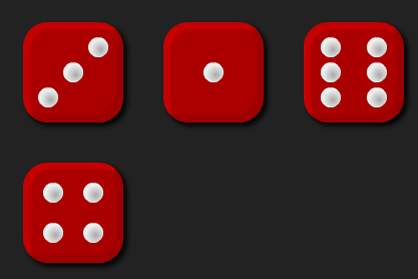#### You may also like### Doplication

We can arrange dots in a similar way to the 5 on a dice and they usually sit quite well into a rectangular shape. How many altogether in this 3 by 5? What happens for other sizes?### Round and Round the Circle

What happens if you join every second point on this circle? How about every third point? Try with different steps and see if you can predict what will happen.### Making Cuboids

Let's say you can only use two different lengths - 2 units and 4 units. Using just these 2 lengths as the edges how many different cuboids can you make?

# Round the Four Dice

## Round the Four DiceThere are four dice, each of them with faces labelled from 1 to 6.
When the dice are rolled they can be combined in 24 different ways to make a four-digit number.
For example, if I roll a 1, 2, 3 and 4 I can combine them to make:
1234, 1243, 1324, 1342, 1423, 1432, 2134, 2143, 2314, 2341, 2413, 2431, 3124, 3142, 3214, 3241, 3412, 3421, 4123, 4132, 4213, 4231, 4312 or 4321.

Now pick four different four-digit numbers from the list and round each of them to the nearest multiple of 1000. For example, 1324 rounds to 1000, 2314 rounds to 2000, 4312 rounds to 4000 and 4123 rounds to 4000.
Do the four four-digit numbers you choose ever all round to the same multiple of 1000?
Do the four four-digit numbers you choose ever round to unique multiples of 1000?

There are some interactive dice that you can use for this problem.

#### Why do this activity?

This activity provides a meaningful task for practising rounding four-digit numbers to the nearest multiple of 1000. It encourages children to record their results, notice patterns and make predictions.

#### Possible approach

A possible starting point is using a number line to remind the class what is meant by rounding.
The interactive dice can be used to model the activity on an interactive whiteboard or can be used by the children as they engage with the task. You may also find that individual dice are useful.

As the task is being modelled, the results should be recorded in a table, perhaps like the one below but going all the way up to the 24th four-digit number:

Numbers rolled 1st four-digit number Rounds to 2nd four-digit number Rounds to 3rd four-digit number Rounds to

1, 2, 3 and 4

1234

1000

2314

2000

4312

4000

This organisation of results will support the children to notice patterns and conjecture about when numbers will round to different multiples of 1000.

Some children may move onto the extension tasks (below).

#### Key questions

Which numbers can we make?
What will they round to? Will they round up or down? Why?
Do they round to the same multiple of 1000? Why? When will this happen? When will it not happen?
Does it make a difference if the digits rolled are unique?

#### Possible extensions

Extension 1: Did the class find examples where some of the four-digit numbers round to the same multiple of 1000? Can they then come up with a rule about when this will happen? When will two round to the same? Or three? Or four?
Extension 2: Did the class find examples where each of the four-digit numbers round to a different multiple of 1000? Why? Why not?
Extension 3: What if you change the numbers on the faces of the dice?
Extension 4: What happens if you always have 0 units and just roll three dice to generate the 10s, 100s and 1000s digits? For example, if I roll 1, 2 and 3, I can make 1230, 1320, 2130, 2310, 3120 or 3210.
Extension 5: What happens if you round to the nearest multiple of 100 instead of the nearest multiple of 1000?

#### Possible support

Copies of the table for children to write the numbers into would be useful.
A number line would also be helpful to support children in reasoning about which whole number is closest to a given multiple of 1000.
Children may like to try Round the Three Dice which focuses on rounding to the nearest hundred.